Learn all Concepts of Chapter 3 Class 10 (with VIDEOS). Check - Linear Equations in 2 Variables - Class 10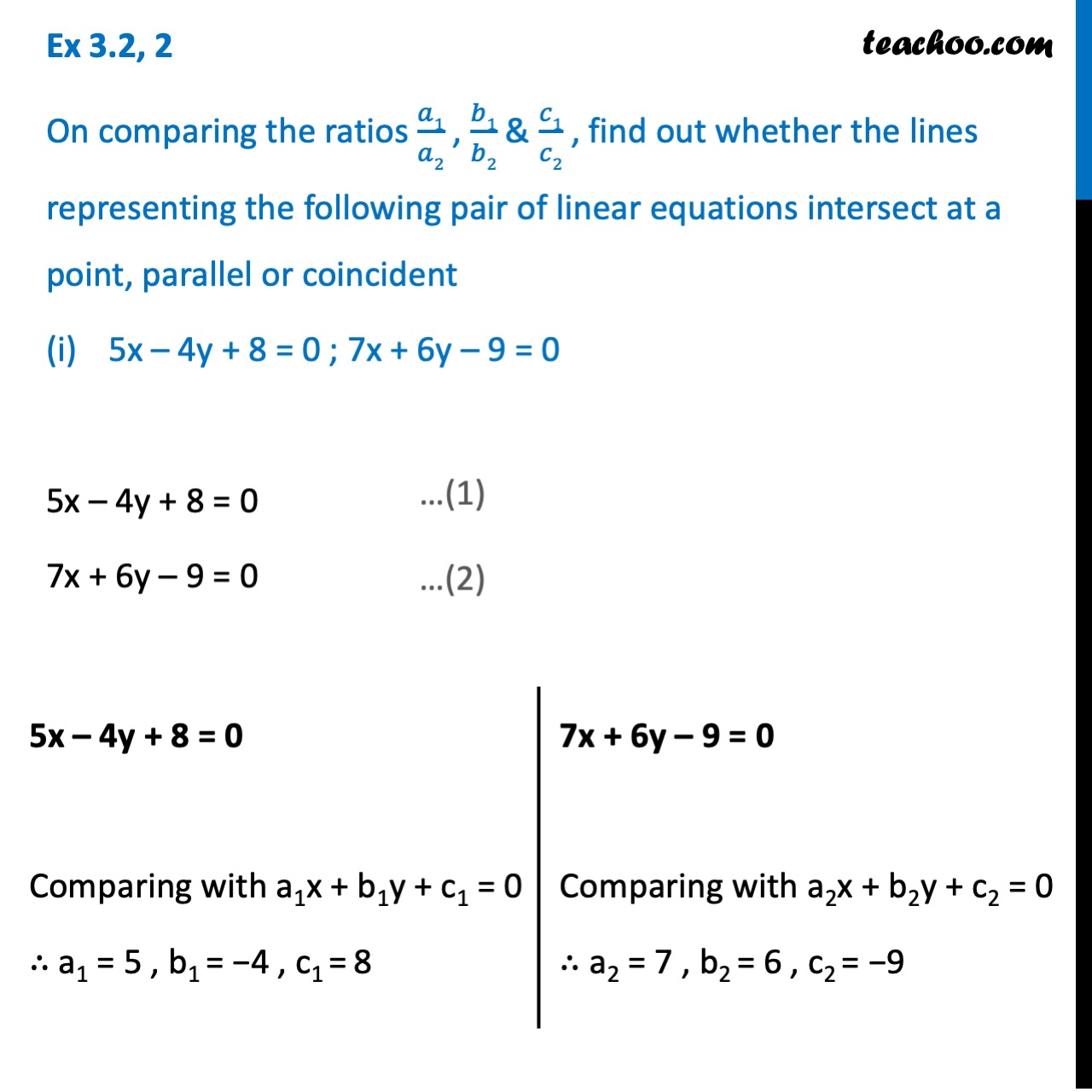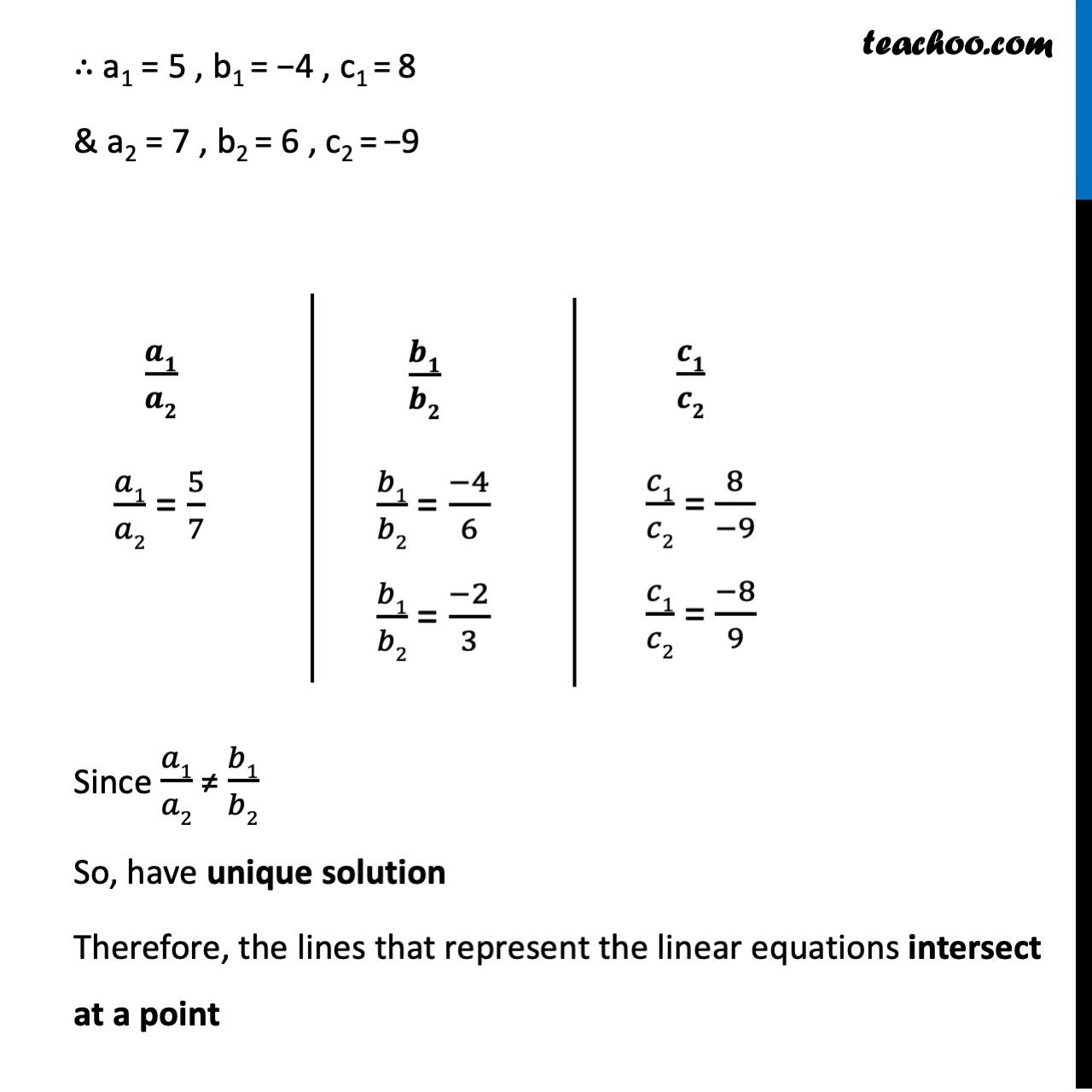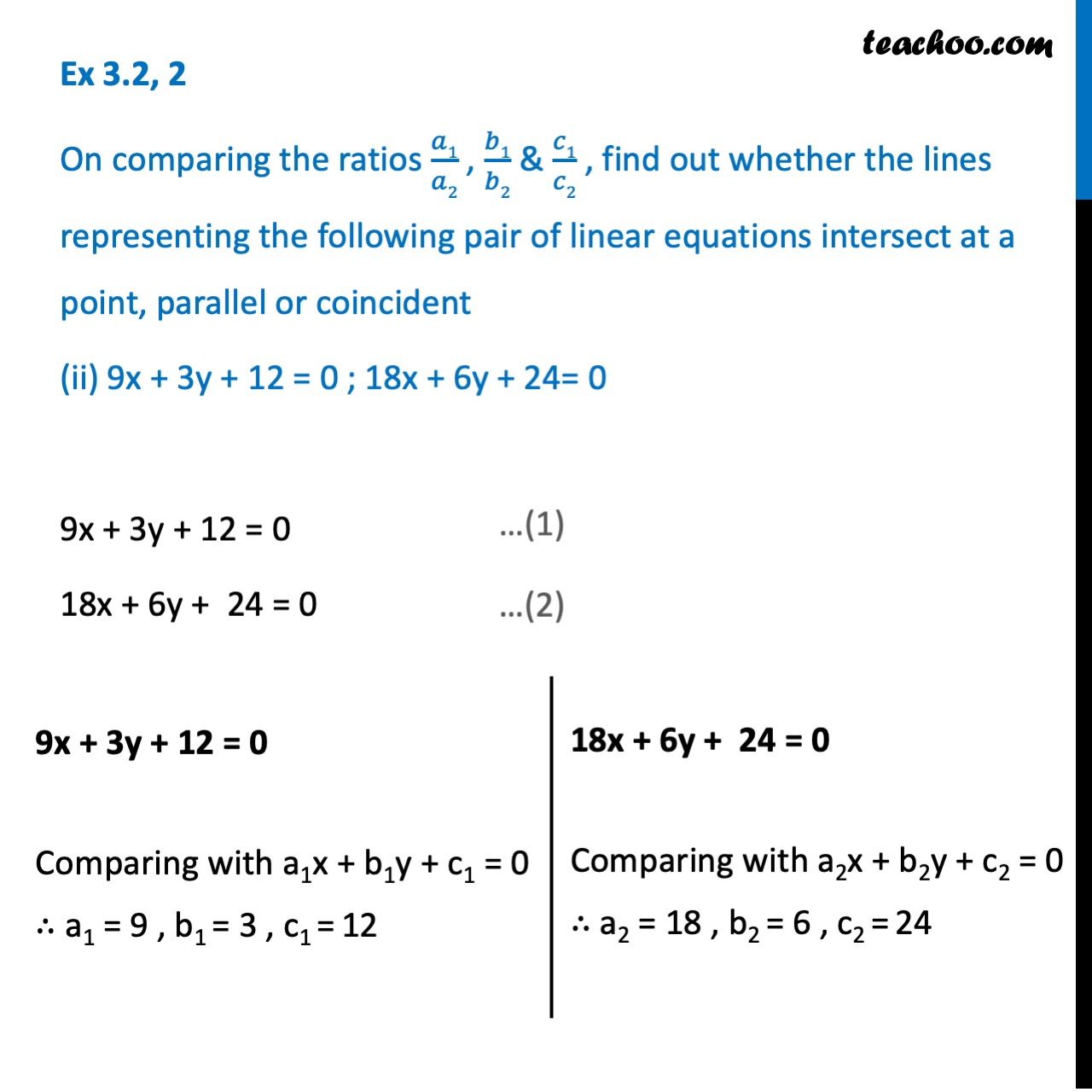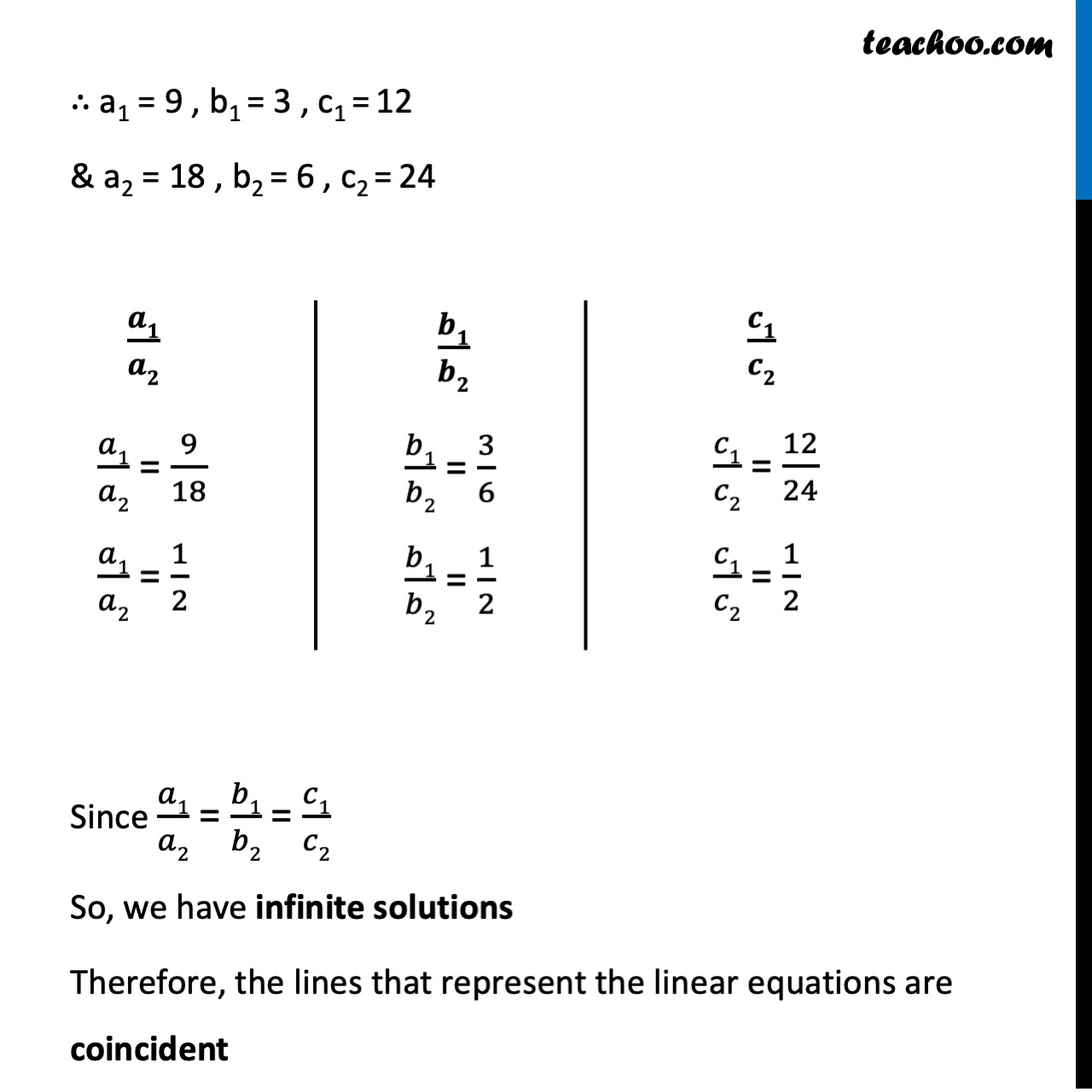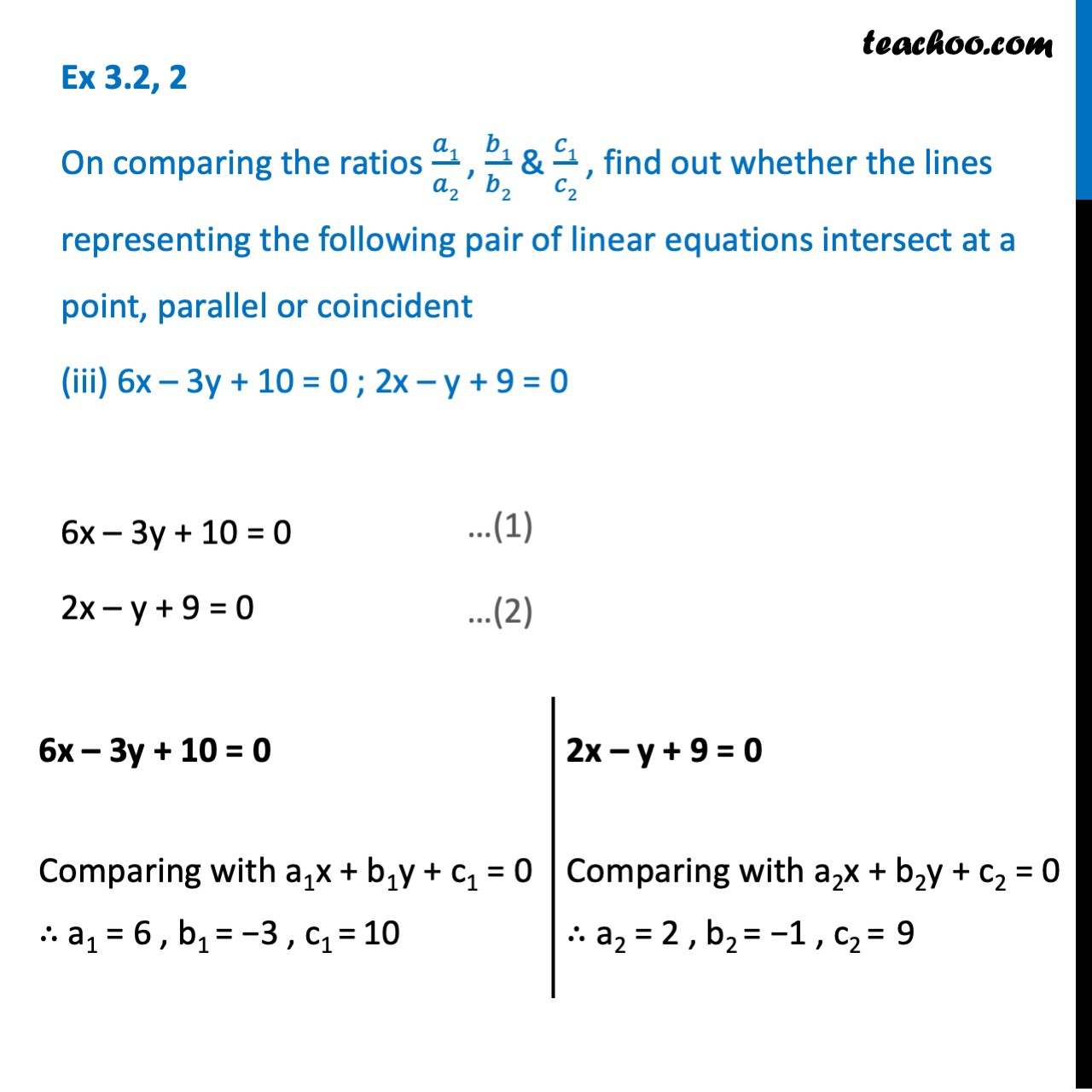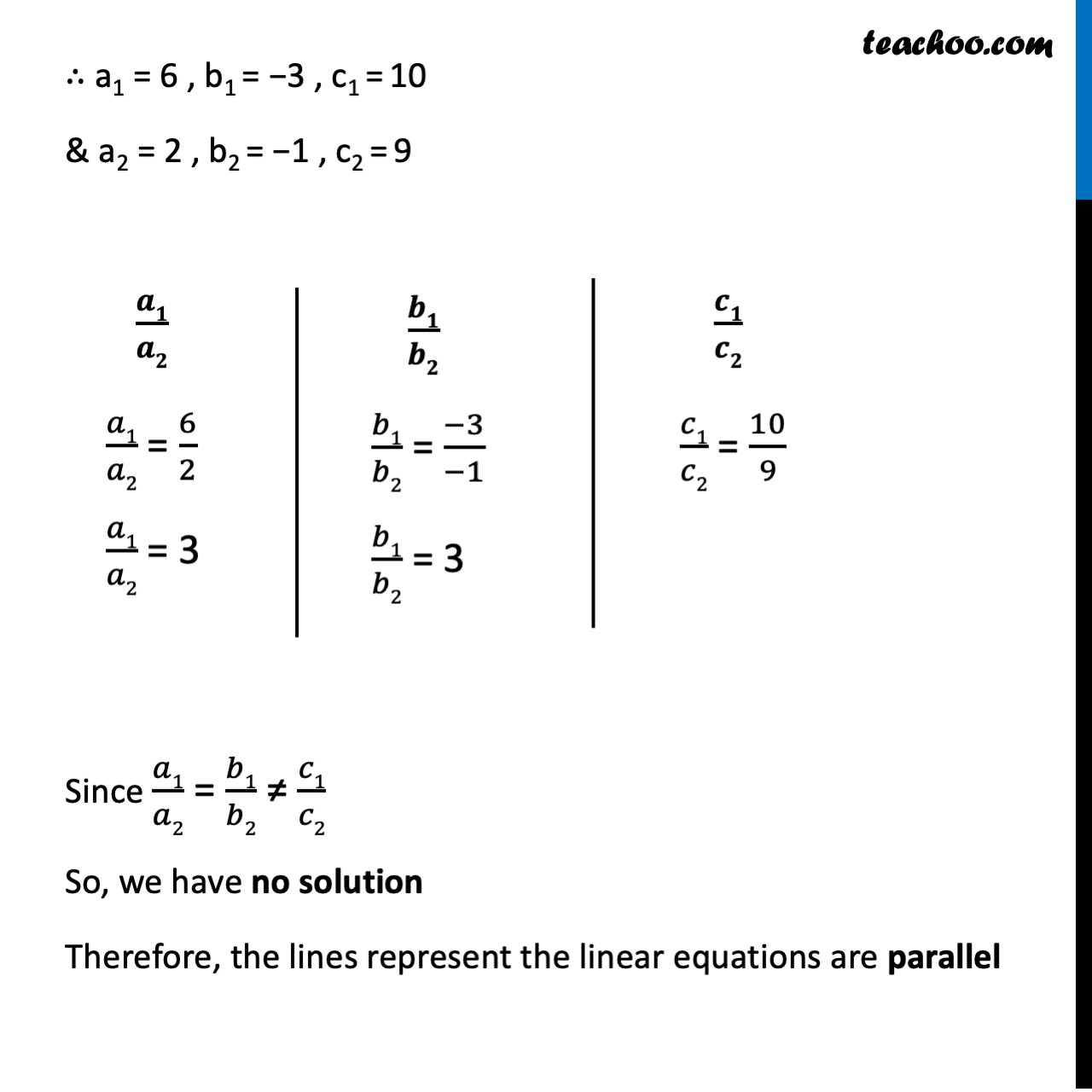1. Chapter 3 Class 10 Pair of Linear Equations in Two Variables
2. Serial order wise
3. Ex 3.2

Transcript

Ex 3.2,2 On comparing the ratios 𝑎1/𝑎2 , 𝑏1/𝑏2 & 𝑐1/𝑐2 , find out whether the lines representing the following pair of linear equations intersect at a point, parallel or coincident 5x – 4y + 8 = 0 ; 7x + 6y – 9 = 0 5x – 4y + 8 = 0 7x + 6y – 9 = 0 ∴ a1 = 5 , b1 = − 4 , c1 = 8 & a2 = 7x , b2 = 6 , c2 = 9 Since 𝑎1/𝑎2 ≠ 𝑏1/𝑏2 We have a unique solution Therefore, the lines that represent the linear equations intersect at a point Ex 3.2,2 On comparing the ratios 𝑎1/𝑎2 , 𝑏1/𝑏2 & 𝑐1/𝑐2 , find out whether the lines representing the following pair of linear equations intersect at a point, parallel or coincident (ii) 9x + 3y + 12 = 0 ; 18x + 6y + 24= 0 9x + 3y + 12 = 0 18x + 6y + 24 = 0 ∴ a1 = 9 , b1 = 3 , c1 = 12 & a2 = 18 , b2 = 6 , c2 = 24 Since 𝑎1/𝑎2 = 𝑏1/𝑏2 = 𝑐1/𝑐2 We have infinite solutions. Therefore, the lines that represent the linear equations are coincident Ex 3.2, 2 On comparing the ratios 𝑎1/𝑎2 , 𝑏1/𝑏2 & 𝑐1/𝑐2 , find out whether the lines representing the following pair of linear equations intersect at a point, parallel or coincident (iii) 6x – 3y + 10 = 0 ; 2x – y + 9 = 0 6x – 3y + 10 = 0 2x – y + 9 = 0 ∴ a1 = 6 , b1 = 3 , c1 = 10 & a2 = 2 , b2 = − 1 , c2 = 9 Since 𝑎1/𝑎2 = 𝑏1/𝑏2 ≠ 𝑐1/𝑐2 We have no solution. Therefore, the lines represent the linear equations are parallel

Ex 3.2